# pandas指南：做更高效的数据科学家

2019/01/25 11:20pandas类似于Python中的Excel：它使用表(即DataFrame)并对数据进行转换，但它还能做更多。

import pandas as pd

pandas包最基本的功能

1、读取数据：

sep变量代表分隔符。因为Excel中的csv分隔符是“;”，因此需要显示它。编码设置为“latin-1”以读取法语字符。nrows=1000表示读取前1000行。skiprows=[2,5]表示在读取文件时将删除第2行和第5行

2、写入数据

data.to_csv('my_new_file.csv', index=None)

index=None将简单地按原样写入数据。如果你不写index=None，会得到额外的行。

3、检查数据：

data.shape
data.describe()

data.loc

data.loc[8, 'column_1']

data.loc[range(4,6)]

pandas的初级功能

1、逻辑运算

data[data['column_1']=='french']

data[(data['column_1']=='french') & (data['year_born']==1990)]

data[(data['column_1']=='french')&(data['year_born']==1990)&(data['city']=='London')]

data[data['column_1'].isin(['french', 'english'])]

2、基本绘图

data['column_numerical'].plot()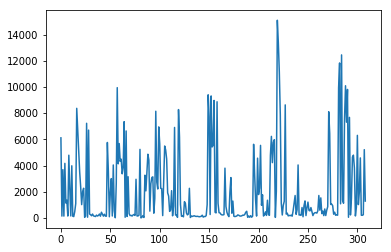data['column_numerical'].hist()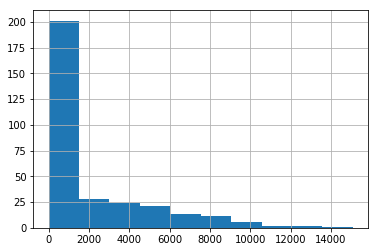%matplotlib inline

3、更新数据

data.loc[8, 'column_1'] = 'english'

data.loc[data['column_1']=='french', 'column_1'] = 'French'

3、pandas的中级功能

1、计算功能

data['column_1'].value_counts()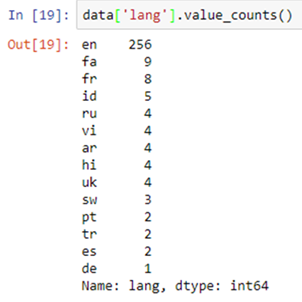2、对全行、全列或所有数据的操作

data['column_1'].map(len)

len()函数应用于“column_1”的每个元素

map()操作将一个函数应用于列的每个元素。

data['column_1'].map(len).map(lambda x : x/100).plot()

pandas的另一个特点是进行链式操作。它可以帮助你在一行代码中执行多个操作，从而更加简单和高效。

data.apply(sum)

.apply()将函数应用于列。

.applymap()将一个函数应用于表(DataFrame)中的所有单元格。

3、tqdm包

from tqdm import tqdm_notebook

tqdm_notebook().pandas()

data['column_1'].progress_map(lambda x : x.count('e'))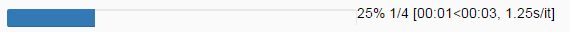4、相关矩阵和散射矩阵

data.corr()
data.corr().applymap(lambda x : int(x*100)/100)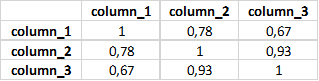pd.plotting.scatter_matrix(data, figsize=(12,8))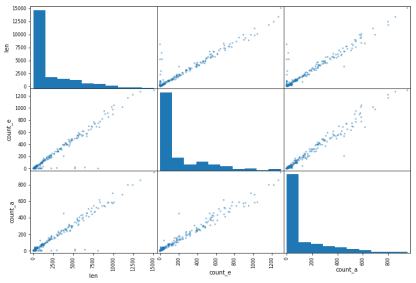pandas的高级功能

1ã行列合并

data.merge(other_data, on=['column_1', 'column_2', 'column_3'])

2、分组

data.groupby('column_1')['column_2'].apply(sum).reset_index()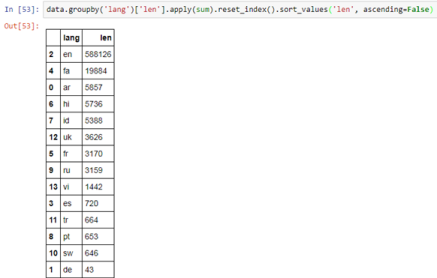3、遍历行

dictionary = {}
for i,row in data.iterrows()：
dictionary[row['column_1']] = row['column_2']

iterrows()循环两个变量：行索引和行(上面代码中的i和row)。

1、 简单易用，隐藏了所有复杂和抽象的计算；

2、非常直观；

3、快速。

0
0 收藏

### 作者的其它热门文章0 评论
0 收藏
0